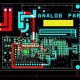# Best Tutorial on Basic Electrical Topics.

In this tutorial, we are going to learn about Best Tutorial on Basic Electrical Topics.

Below are common topics for electrical engineering.

1. Current
2. Voltage
3. Resistance
4. Electric Power
5. Power Efficiency
6. Energy Efficiency
• Current
• It is the rate of flow of electric charge in electrical filed, generally in electrical circuit.
• Current is same as it like using water pipe analogy, the electrical current as water which flow in a pipe.
• The current is measured in ampere.

I = dQ/dT

Here is the I is the current in amps (A).

Q is the electric charge in coulombs (C).

T is the time in second (s).

Types of Current – As per below image can see there is metal where the charge carriers (electron) are negative.

• Conventional Current – It is defined as moving in the same direction as the positive (holes) charge flow.
• Electrical Current. – It is defined as moving in the same direction as the negative (electrons) charge flow.

Electrical Voltage –

• It is defined as electrical potential difference between two points of an electrical field.
• The voltage is measured in Volts (v).
• Example like that using water pipe analogy, we can visualize the voltage as height difference which makes the water flow down.
• In an electrical circuit, the electrical voltage V is equal to the energy consumption E divided by the electric charge Q.

V = E/Q

Here V is the voltage measured in volts (V).

E is the energy measured in joules (J).

Q is the electric charge measured in coulombs (C).

VAB = Q2 – Q1

Resistance –

The resistance of a conductor is resistivity of the conductor’s material times the conductor’s length divided by the conductor’s cross sectional area.

R = ϸ x L/A

R is the resistance in ohms (Ὠ).

ϸ is the resistivity in ohm – meter (Ὠ x m).

L is the length of the conductor in meter (m).

A is the cross sectional area of the conduction in square meters.

Electric Power –

The electric power P is the equal to the energy consumption E divided by the consumption time t.

P = E/t

P is the electric power in watt (W).

E is the energy consumption in joule (J).

T is time in seconds (S).

Electric Power Calculation Formula:

P = VXI

P = I2 X R

P = V2 / R

P is the electric power in watt (W).

V is the voltage in volts (V).

I is the current in amp (A).

R is resistance in ohms (Ὠ).

Electrical Power Efficiency –

Power Efficiency is defined as the ratio of the output power divided by the input power:

η = 100. POUT / PIN

η is the Efficiency in percent (%).

PIN is the input power consumption in watts (W).

POUT is the output power consumption in watts (W).

Energy Efficiency –

It is defined as the ratio of the output energy divided by input energy:

η = 100. EOUT / EIN

η is the Efficiency in percent (%).

EIN is the input energy consumed in joule (J).

EOUT is the output energy consumed in joule (J).